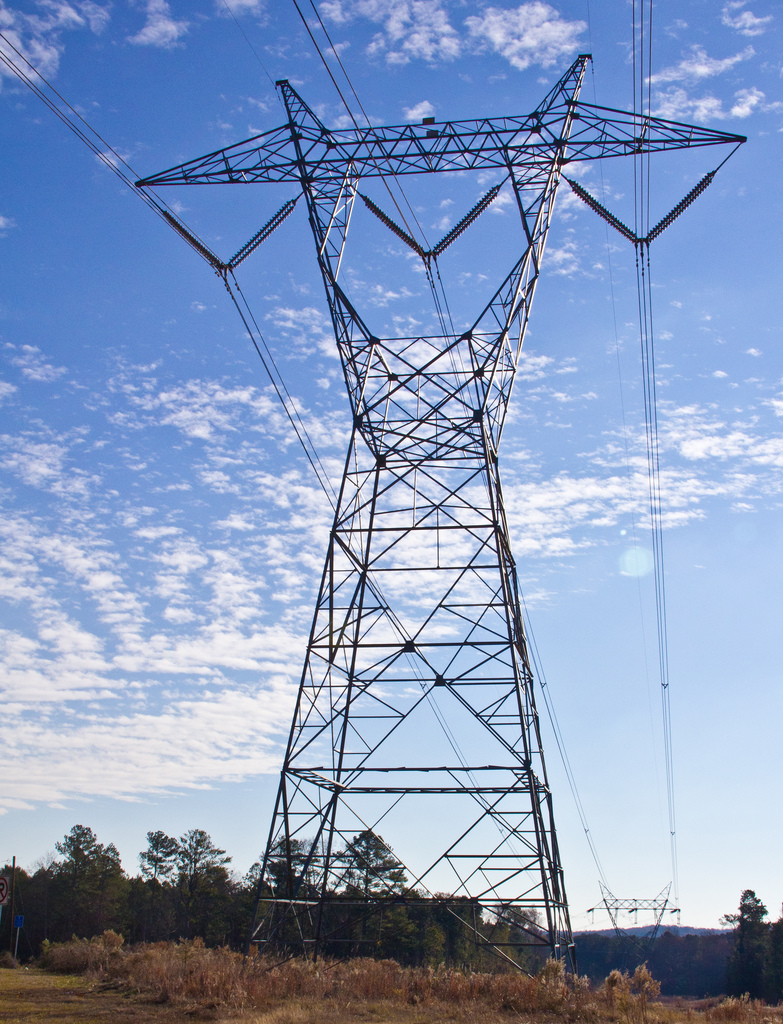# MVARs Relationship with Grid and Loads

### What are the Components?

VARs are probably the most confusing portion of the Grid and Loads relationship. First, thing to understand is that it only applies to Alternating Current (AC). In theory the most basic description needed for VARs  can be derived from the Power Triangle (right). Power in any direction has the base components of Voltage and Current. But, Reactive power contains an additional component – reactance. In AC systems there are 3 types of resistance or more commonly called impedance: Real Resistance and Non-Real Resistance called Reactance which is attributed to inductive resistance, and capacitive resistance.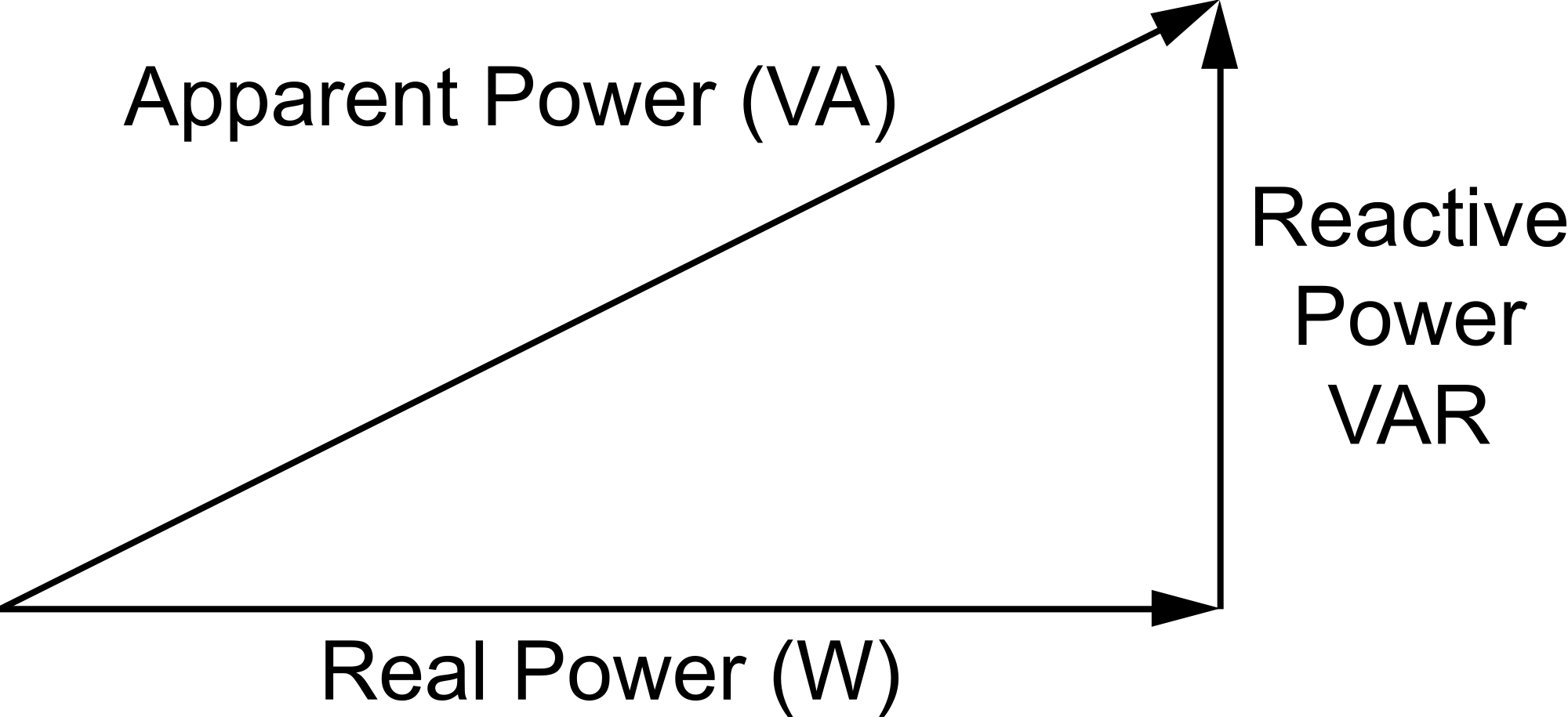To describe a metaphor that represents this relationship. Imagine a bucket that is filled with water. There are only two actions that can be taken. Pour more water in with a hose, or suck water out with a water pump. The water level is a measure of reactive impedance. The hose is voltage and the pump is current. As the hose pushes water into the bucket, the water level rises. Signifying an increase in capacative load. As the pump sucks water out the water level drops, signifying a decrease in phase angle between the current and voltage. The impedance would technically be how hard it is to fill the bucket. And real resistance is the middle of the bucket, with 0 impedance. This is very crud illistration but is makes the point. If you have an increase in distance between the voltage signal and current signal you have an increase in impedance. {review and delete if necessary}

A one sentence, full encompassment of MVARs could be defined as power that results from the interaction between current and voltage phases due to capacitance and inductance on AC systems. I micro-level of understanding is not really necessary for the practical scenarios we will describe later.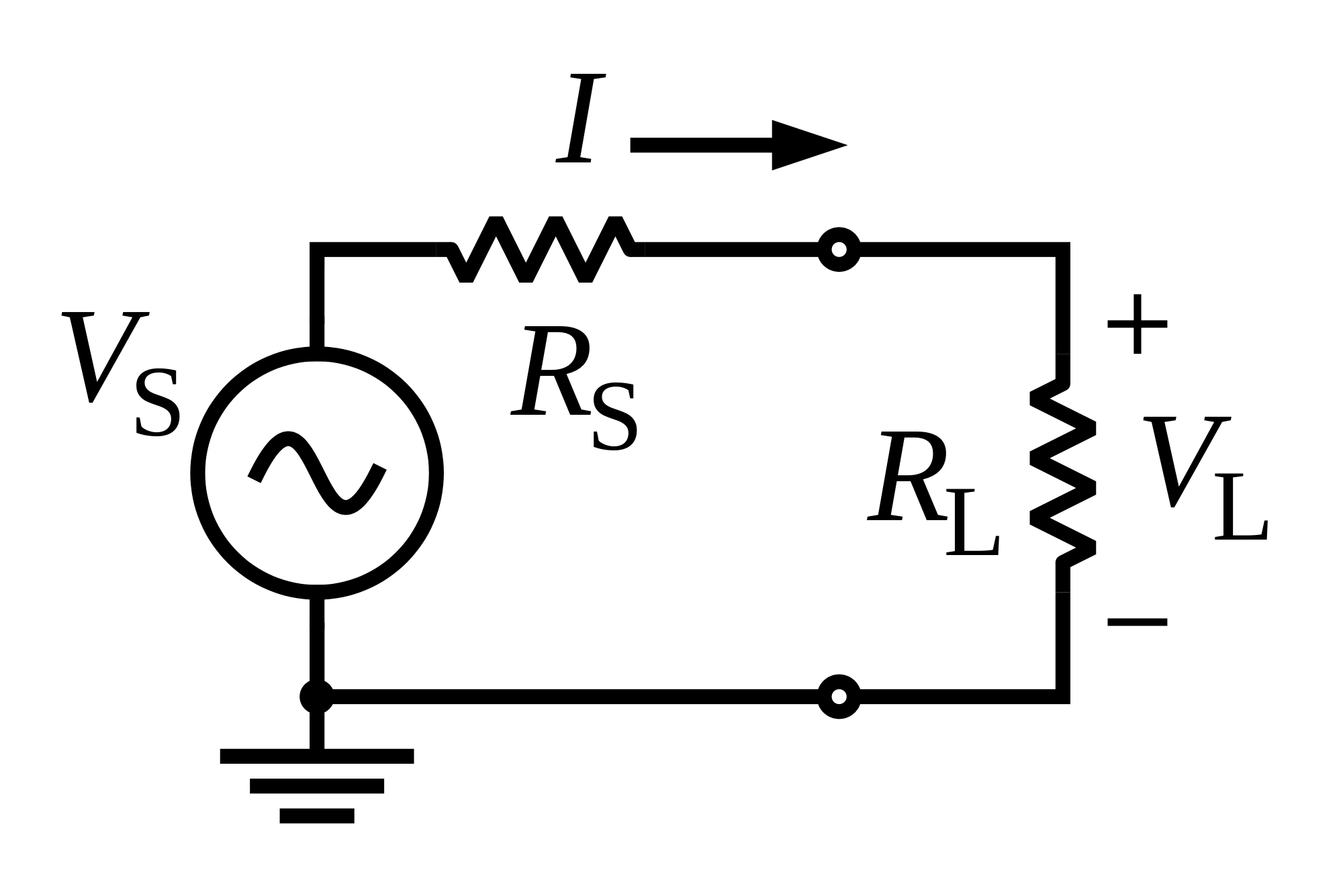LOAD in a system represents the pull of the system. Essentially, the load is the resistance between the power source and ground. The load will control power output of the power source. Load determines the current amount needed, and voltage represents a level of push needed to obtain the necessary power. The voltage however, is typically thought of as a constant figure in transmission. But, in reality Voltage fluctuates as much as the power is demanded. But it is controlled to a level of tolerance. There is a boundary that voltage is not allowed to cross due to the required voltage levels of the load. We must remember most machines, motors, and load is voltage operated. {There have been some devices created that operate on current, but I am unfamiliar with those systems. }

A one sentence, full encompassment of Load could be defined as a power dissipater that controls the amount of power generated at the source. From a power prospective, load is just the element of the circuit that uses the power. The load tells the circuit how much current is needed. If there is no resistance in the circuit, then there is and increase in current. If current increases, then power increases. If power increases, then the power source will generate more.

GRID refers to an electrical distribution system composed of components that support a means of transferring energy from point to point. Transformers, capacitors, cables, protection and correction devices enable the seamless transferal of Alternating Current from Source to Load. That is a basic understanding the most of the readers will have. However, there are many other components offering additional system support that I didn’t mention.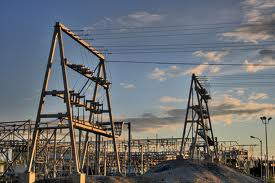### What are the modes of change?

There are 5 main electrical abstracts that effect system performance. In a high-level summary we can consider the following:

1.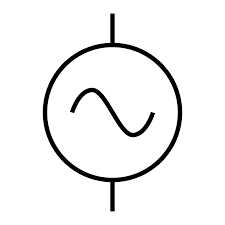Voltage is the pusher. Typically the voltage is stepped up through a transformer to high voltage levels to increase efficiency. The voltage is tapped to a voltage level to supply current grid conditions. Nominal voltage tolerance will demand a small window of change. The goal is to keep the voltage near a constant level.
2.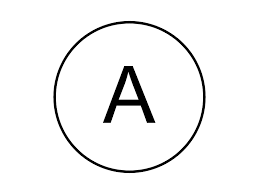Current is the puller. Current is a result of a complete circuit that has an electrical resistance and a voltage.
3.Resistance is an electrical element that acts as a restrictor to electron magnetic forces.
4.Capacitance
is the ability of the circuit to store charge.
5.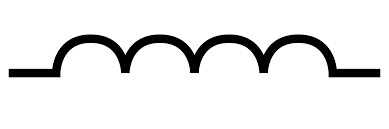Inductance is the ability of the circuit that acts as a resistance to change in current.

Essentially, there are many elements in a transmission line that affect each other and are directly related as seen in the table to the right.

### How does one affect the other?

By creating some scenarios we can start to understand relationships between the modes of change on a Grid. To keep is as simple for understanding as possible some of the fluff is left off. I will emphasis the VAR relationship.

Scenario 1: What happens if Resistive load increases?

Equation Explanation VAR Reaction
I=V/R As resistance increases current decreases, as long as voltage is constant. Negative

Scenario 2: What happens as Inductance increases?

Equation Explanation VAR Reaction
Z=√(R^2+X^2 )

and

I = V/Z

If inductance increases the magnitude of impedance increases. Therefore, if voltage is held constant then current would decrease yet again. Also, think about the power triangle and what is happening as you add inductance. Negative

Scenario 3: What happens if Voltage increases in the Grid?

Equation Explanation VAR Reaction
V=IR

and

Q=VI*sin(∅)

As voltage increases current increases, respective to no change in load.

As Voltage or Current increases so does Reactive Power.

Positive

Scenario 4: What happens in a Voltage Regulator for each of the previous scenarios? (Assume the VR is controlling generator source)

Scenario Explanation
1 If load increases on the grid then voltage will want to increase. However, the VR wants to keep voltage within its expectable band, so it will only allow it to increase slightly. The VR will then change the field excitation to decrease generator output voltage.
2 Same as above
3 Same as above

As you can see the voltage regulator just wants to control voltage. However, some VR contain a reactive current compensator that changes reactance output. But, in the scale of the grid, it is a grid effort to compensate on a large scale.

### Conclusion

The fundamental Understanding of MVARs Relationship with Grid and Loads is really the power component attributed to inductance and capacitance in the system. It can directly influence grid conditions, and effect loads. If the imaginary component of the grid got out of hand, one could see the entire grid could be taken down. As seen in the scenarios above, one electrical abstract effects another. The main take away I people to take away from this article are what is VARs and how does it relate.